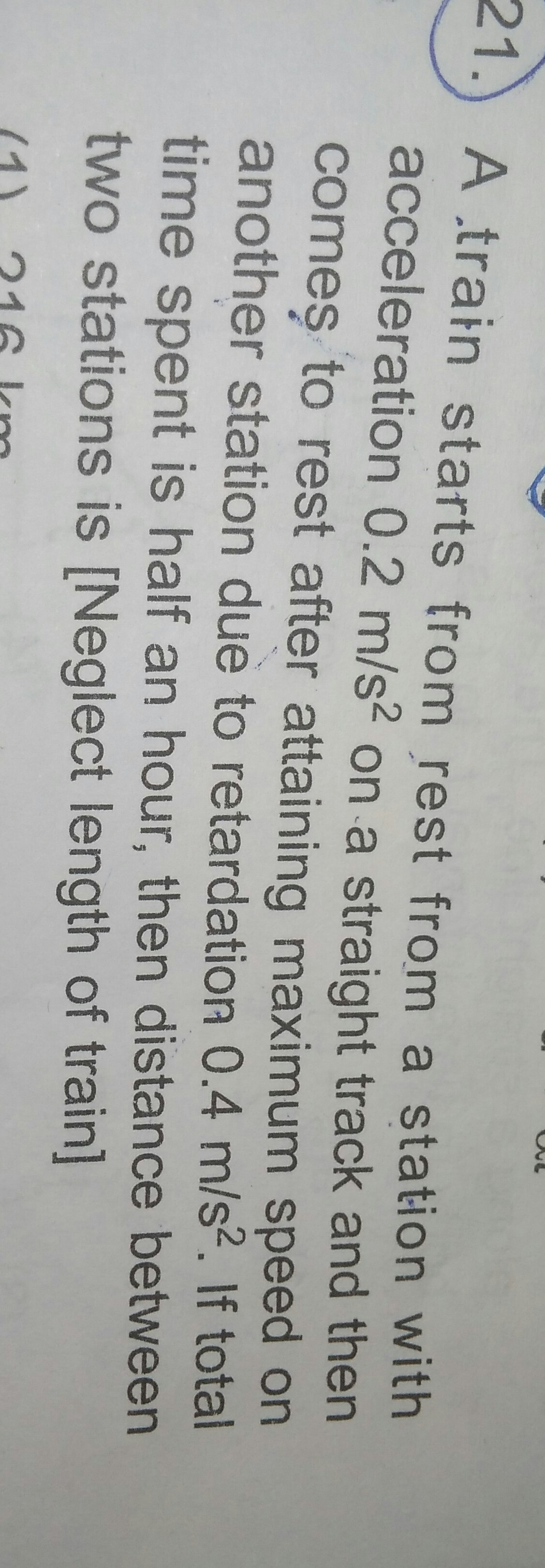# a train starts from rest from a station with accleration 0.2 on a staight track and then comes to rest after attaning maximum speed on another station due to retardation 0.4 ..if total time spent is half an hour, then distance between two stations is {neglect length of train}...4 years ago
Dear Shubham

Case I:

Train accelerates with a = 0.2 m/s, t = t1and v= v1

v=u + at and v1=0.2tor t1= 0.2 v1 /2 …(1)

s=ut+(1/2)at2

Case II:

v2=v1+a2t2(train is retarding and s =s1)

s1=1/2 x 0.2 x t12

v2=v1+a2t2

0 = v1+0.4t2

t2=–v1/0.4 …(2)

s2=v1t2 +(1 /2) x a2t22

t=t1+t2 = 30 min = 1800

t1=1200 secs and t2 = 600 secs

substituting a, v and t in (1) and (3), we get

s1 = 144 km and s2= 72 km

Total distance s= s2+ s2

s = 216km

Regards

Arun (askIITians forum expert)